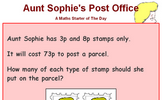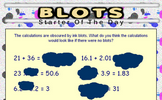# Indices

### Term 2 starting in week 6 :: Estimated time: 1 weeks

• Adding and subtracting expressions with indices
• Simplifying algebraic expressions by multiplying indices
• Simplifying algebraic expressions by dividing indices
• Using the addition law for indices
• Using the addition and subtraction law for indices
For higher-attaining pupils:
• Exploring powers of powers

This page should remember your ticks from one visit to the next for a period of time. It does this by using Local Storage so the information is saved only on the computer you are working on right now.

## Lesson Starters

Here are some suggestions for whole-class, projectable resources which can be used at the beginnings of each lesson in this block.

### 1st Lesson#### Aunt Sophie's Post Office

Work out the number of stamps needed to post a parcel.

### 2nd Lesson#### Blots

The calculations are obscured by ink blots. What do you think the calculations would look like if there were no blots?

### 3rd Lesson#### Exponential Error

This lesson starter presents a number of statements about indices and pupils are asked if they can spot the mistake.

Some of the Starters above are to reinforce concepts learnt, others are to introduce new ideas while others are on unrelated topics designed for retrieval practice or and opportunity to develop problem-solving skills.

White Rose ResourcesEnd of block assessments provide a quick progress check at the end of each block of learning to make sure students have understood the content covered. This Scheme of Learning was produced by White Rose Maths and is used here with permission granted on 30th June 2021.For All: